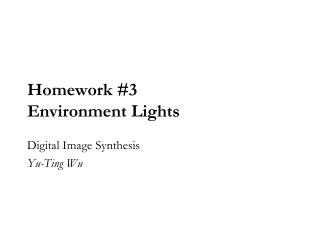# Homework #3 Environment Lights - PowerPoint PPT PresentationDownload PresentationHomework #3 Environment Lights

Homework #3 Environment LightsDownload Presentation## Homework #3 Environment Lights

- - - - - - - - - - - - - - - - - - - - - - - - - - - E N D - - - - - - - - - - - - - - - - - - - - - - - - - - -
##### Presentation Transcript

1. Homework #3Environment Lights Digital Image Synthesis Yu-Ting Wu

2. Project description • Pbrt uses run-time importance sampling to render scenes with environment lights • The details will be given when talking about Monte Carlo integration • In this homework, you are asked to provide an alternative approach The brighter pixels (lights) have higher probability to be sampled

3. Project description • Represent the environment map with a set of point (directional) lights A light probe image is subdivided into 64 equal-energy regions. A point light is created for each region at its centroid.

4. Project description • Implement the “MedianCut” algorithm in pbrt • There are three functions you should modify: • Constructor • Do MedianCut algorithm to generate a set of lights with roughly equal energy • Sample_L • When pbrt asks a sample from environment light, uniformly select one from all lights and return its direction, intensity, and PDF • IsDeltaLight • Tell pbrt whether this light can be sampled or not? • For any other member functions, you can leave them alone at this time

5. Importance sampling • In rendering, one important question is how to determine the sample distributions • Importance sampling: • If the shape of sampling distribution is close to the shape of integrand, variance is reduced • In our case Sample_L Sample_f

6. Multiple importance sampling • Sample light or sample BRDF only cannot adapt to all configurations • Multiple importance sampling: • Generate one sample using Sample_L and another using Sample_f, then combine the sample results • Better than either of the two strategies alone Sample Light Sample BRDF Multiple Importance Sampling

7. Multiple importance sampling • Codes for estimating direct lighting in pbrt:

8. Multiple importance sampling

9. Multiple importance sampling • IsDeltaLight • In homework #3, since we transform the environment to a set of directional lights which cannot be sampled, we should return true to avoid “BRDF importance sampling” • Sample_L • Pbrt only asks one sample at a time. • In homework #3, all lights have roughly equal energy. • We can simply random choose one from n lights and return PDF with 1/n

10. FAQ • The rendered images have different radiance scale to the reference ones • Each pixel in the environment map represents an area on unit sphere • Remember to scale the light intensity with the areas (solid angles) width  2PI w h HeightPI

11. Related papers Variance Minimization Light Probe Sampling K. Viriyothai and P. Debevec SIGGRAPH 2009 Poster Structured Importance Sampling of Environment Maps S. Agarwal, R. Ramamoorthi, S. Belongie, and H. W. Jensen SIGGRAPH 2002 Efficient Illumination by High Dynamic Range Images T. Kolling and A. Keller EGSR 2003 Fast Hierarchical Importance Sampling with Blue Noise Properties V. Ostromoukhov, C. Donohue, and P. –M. Hodoin SIGGRAPH 2004